# NCERT Solutions For Class 12 Maths Chapter 9 Exercise 9.3

## Chapter 9 Ex.9.3 Question 1

Form a differential equation representing the given family of curves by eliminating arbitrary constants $$a$$ and $$b$$.

$$\frac{x}{a} + \frac{y}{b} = 1$$

### Solution

\begin{align}&\frac{x}{a} + \frac{y}{b} = 1\\ &\Rightarrow \; \frac{1}{a} + \frac{1}{b}\frac{{dy}}{{dx}} = 0\\& \Rightarrow \; \frac{1}{a} + \frac{1}{b}y' = 0\\ &\Rightarrow \; 0 + \frac{1}{b}y'' = 0\\& \Rightarrow \; \frac{1}{b}y'' = 0\\ &\Rightarrow \; y'' = 0\end{align}

Thus, the differential equation of the given curve is $$y'' = 0$$.

## Chapter 9 Ex.9.3 Question 2

Form a differential equation representing the given family of curves by eliminating arbitrary constants $$a$$ and $$b$$.

$${y^2} = a\left( {{b^2} - {x^2}} \right)$$

### Solution

\begin{align}&{y^2} = a\left( {{b^2} - {x^2}} \right)\\& \Rightarrow \; 2y\frac{{dy}}{{dx}} = a\left( { - 2x} \right)\\ &\Rightarrow \; 2yy' = - 2ax\\ &\Rightarrow \; yy' = - ax\\ &\Rightarrow \; y'.y' + yy'' = - a\\ &\Rightarrow \; \frac{{{{\left( {y'} \right)}^2} + yy''}}{{yy'}} = \frac{{ - a}}{{ - ax}}\\ &\Rightarrow \; xyy'' + x{\left( {y'} \right)^2} - yy' = 0\end{align}

Thus, the differential equation of the given curve is $$xyy'' + x{\left( {y'} \right)^2} - yy' = 0$$.

## Chapter 9 Ex.9.3 Question 3

Form a differential equation representing the given family of curves by eliminating arbitrary constants $$a$$ and $$b$$.

$$y = a{e^{3x}} + b{e^{ - 2x}}$$

### Solution

\begin{align}&y = a{e^{3x}} + b{e^{ - 2x}}\\&y' = 3a{e^{3x}} - 2b{e^{ - 2x}} \qquad \ldots \left( 1 \right)\\&y'' = 9a{e^{3x}} + 4b{e^{ - 2x}} \qquad \ldots \left( 2 \right)\end{align}

Subtracting $$\left( 1 \right)$$ from $$\left( 2 \right)$$

\begin{align}&y'' - y' = 9a{e^{3x}} + 4b{e^{ - 2x}} - 3a{e^{3x}} + 2b{e^{ - 2x}} \hfill \\&y'' - y' = 6a{e^{3x}} + 6b{e^{ - 2x}} \hfill \\&y'' - y' = 6\left( {a{e^{3x}} + b{e^{ - 2x}}} \right) \hfill \\&y'' - y' = 6y \qquad \left( {\because y = a{e^{3x}} + b{e^{ - 2x}}} \right) \hfill \\&y'' - y' - 6y = 0 \hfill \\\end{align}

Thus, the differential equation of the given curve is $$y'' - y' - 6y = 0$$.

## Chapter 9 Ex.9.3 Question 4

Form a differential equation representing the given family of curves by eliminating arbitrary constants $$a$$ and $$b$$.

$$y = {e^{2x}}\left( {a + bx} \right)$$

### Solution

\begin{align}&y = {e^{2x}}\left( {a + bx} \right)\\&y' = 2{e^{2x}}\left( {a + bx} \right) + {e^{2x}}b\\ &\Rightarrow \; y' = {e^{2x}}\left( {2a + 2bx + b} \right)\\&y' - 2y = {e^{2x}}\left( {2a + 2bx + b} \right) - {e^{2x}}\left( {2a + 2bx} \right)\\& \Rightarrow \; y' - 2y = b{e^{2x}} \qquad \qquad \ldots \left( 1 \right)\\ &\Rightarrow \; y'' - 2y' = 2b{e^{2x}} \qquad \quad \ldots \left( 2 \right)\end{align}

Dividing $$\left( 2 \right)$$ by $$\left( 1 \right)$$

\begin{align}&\frac{{y'' - 2y'}}{{y' - 2y}} = 2\\ &\Rightarrow \; y'' - 2y' = 2y' - 4y\\ &\Rightarrow \; y'' - 4y' + 4y = 0\end{align}

Thus, the differential equation of the given curve is $$y'' - 4y' + 4y = 0$$.

## Chapter 9 Ex.9.3 Question 5

Form a differential equation representing the given family of curves by eliminating arbitrary constants $$a$$ and $$b$$.

$$y = {e^{3x}}\left( {a\cos x + b\sin x} \right)$$

### Solution

\begin{align}&y = {e^x}\left( {a\cos x + b\sin x} \right)\\&y' = {e^x}\left( {a\cos x + b\sin x} \right) + {e^x}\left( { - a\sin x + b\cos x} \right)\\ &\Rightarrow \; y' = {e^x}\left[ {\left( {a + b} \right)\cos x - \left( {a - b} \right)\sin x} \right]\\ &\Rightarrow \; y'' = {e^x}\left[ {\left( {a + b} \right)\cos x - \left( {a - b} \right)\sin x} \right] \\&\qquad+ {e^x}\left[ { - \left( {a + b} \right)\sin x - \left( {a - b} \right)\cos x} \right]\\&y'' = {e^x}\left[ {2b\cos x - 2a\sin x} \right]\\&y'' = 2{e^x}\left( {b\cos x - a\sin x} \right)\\ &\Rightarrow \; \frac{{y''}}{2} = {e^x}\left( {b\cos x - a\sin x} \right)\\&\frac{{y''}}{2} + y = {e^x}\left( {b\cos x - a\sin x} \right) + {e^x}\left( {a\cos x + b\sin x} \right)\\&y + \frac{{y''}}{2} = {e^x}\left[ {\left( {a + b} \right)\cos x - \left( {a - b} \right)\sin x} \right]\\ &\Rightarrow \; y + \frac{{y''}}{2} = y'\\& \Rightarrow \; 2y + y'' = 2y'\\& \Rightarrow \; y'' - 2y' + 2y = 0\end{align}

Thus, the differential equation of the given curve is $$y'' - 2y' + 2y = 0$$.

## Chapter 9 Ex.9.3 Question 6

Form the differential equation of the family of circles touching the $$y$$-axis at origin.

### Solution

Centre of circle touching the $$y$$-axis at origin lies on the $$x$$-axis.

Let $$\left( {a,0} \right)$$ be the centre of the circle.

Since it touches the $$y$$-axis at origin, its radius is $$a$$.

Equation of the circle with centre $$\left( {a,0} \right)$$ and radius $$a$$ is

\begin{align}&{\left( {x - a} \right)^2} + {y^2} = {a^2}\\ &\Rightarrow \; {x^2} + {y^2} = 2ax \qquad \ldots \left( 1 \right)\end{align}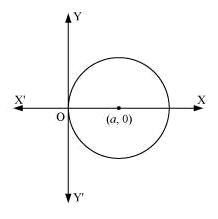Differentiating equation $$\left( 1 \right)$$ with respect to $$x$$, we get:

\begin{align}&2x + 2yy' = 2a\\& \Rightarrow \; x + yy' = a\end{align}

Putting the value of $$a$$ in equation $$\left( 1 \right)$$

\begin{align} &\Rightarrow \; {x^2} + {y^2} = 2\left( {x + yy'} \right)x\\& \Rightarrow \; 2xyy' + {x^2} = {y^2}\end{align}

## Chapter 9 Ex.9.3 Question 7

Form the differential equation of the family of parabolas having vertex at origin and axis along positive $$y$$-axis.

### Solution

The equation of the parabola having the vertex at origin and the axis along the positive $$y$$-axis is

$${x^2} = 4ay \qquad \ldots \left( 1 \right)$$.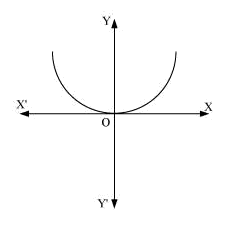Differentiating equation $$\left( 1 \right)$$ with respect to $$x$$, we get:

\begin{align}2x &= 4ay'\\ &\Rightarrow \; \frac{{2x}}{{{x^2}}} = \frac{{4ay'}}{{4ay}}\\& \Rightarrow \; \frac{2}{x} = \frac{{y'}}{y}\\& \Rightarrow \; xy' = 2y\\ &\Rightarrow \; xy' - 2y = 0\end{align}

## Chapter 9 Ex.9.3 Question 8

Form the differential equation of the family of ellipses having foci on-axis and centre at origin.

### Solution

The equation of the family of ellipses having foci on the $$y$$-axis and the centre at origin is as follows:

$$\frac{{{x^2}}}{{{b^2}}} + \frac{{{y^2}}}{{{a^2}}} = 1 \qquad \ldots \left( 1 \right)$$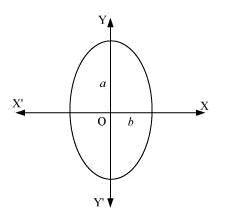Differentiating equation $$(1)$$ with respect to $$x$$, we get:

\begin{align}&\frac{{2x}}{{{b^2}}} + \frac{{2yy'}}{{{a^2}}} = 0\\ &\Rightarrow \; \frac{x}{{{b^2}}} + \frac{{yy'}}{{{a^2}}} = 0 \qquad \dots \left( 2 \right)\end{align}

Differentiating equation $$\left( 2 \right)$$ with respect to $$x$$, we get:

\begin{align}&\frac{1}{{{b^2}}} + \frac{{y'.y' + y.y''}}{{{a^2}}} = 0\\ &\Rightarrow \; \frac{1}{{{b^2}}} + \frac{1}{{{a^2}}}\left( {{{y'}^2} + yy''} \right) = 0\\ &\Rightarrow \; \frac{1}{{{b^2}}} = - \frac{1}{{{a^2}}}\left( {{{y'}^2} + yy''} \right)\end{align}

Substituting this value in equation $$\left( 2 \right)$$, we get:

\begin{align} &\Rightarrow \; x\left[ { - \frac{1}{{{a^2}}}\left( {{{y'}^2} + yy''} \right)} \right] + \frac{{yy'}}{{{a^2}}} = 0\\& \Rightarrow \; - x{{y'}^2} - xyy'' + yy' = 0\\ &\Rightarrow \; xyy'' + x{{y'}^2} - yy' = 0\end{align}

## Chapter 9 Ex.9.3 Question 9

Form the differential equation of the family of hyperbolas having foci on $$x$$-axis and centre at origin.

### Solution

The equation of the family of hyperbolas with the centre at origin and foci along the $$x$$-axis is

$$\frac{{{x^2}}}{{{b^2}}} + \frac{{{y^2}}}{{{a^2}}} = 1 \qquad \ldots \left( 1 \right)$$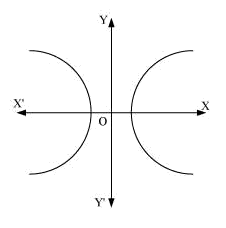Differentiating equation $$\left( 1 \right)$$ with respect to $$x$$, we get:

\begin{align}&\frac{{2x}}{{{b^2}}} + \frac{{2yy'}}{{{a^2}}} = 0\\ &\Rightarrow \; \frac{x}{{{b^2}}} + \frac{{yy'}}{{{a^2}}} = 0 \qquad \ldots \left( 2 \right)\end{align}

Differentiating equation $$\left( 2 \right)$$ with respect to $$x$$, we get:

\begin{align}&\frac{1}{{{b^2}}} + \frac{{y'.y' + y.y''}}{{{a^2}}} = 0\\&\frac{1}{{{b^2}}} = - \frac{{y'.y' + y.y''}}{{{a^2}}}\end{align}

Substituting this value in equation $$\left( 2 \right)$$, we get:

\begin{align}x\left[ { - \frac{{y'.y' + y.y''}}{{{a^2}}}} \right] + \frac{{yy'}}{{{a^2}}} &= 0\\\frac{x}{{{a^2}}}\left[ {y'.y' + y.y''} \right] - \frac{{yy'}}{{{a^2}}} &= 0\\ \Rightarrow \; x{{y'}^2} + xyy'' - yy' &= 0\\ \Rightarrow \; xyy'' + x{{y'}^2} - yy' &= 0\end{align}

## Chapter 9 Ex.9.3 Question 10

Form the differential equation of the family of circles having centre on $$y$$-axis and radius $$3$$ units.

### Solution

Let centre of the circle on $$y$$-axis be $$\left( {0,b} \right)$$.

\begin{align}&{x^2} + {\left( {y - b} \right)^2} = {3^2}\\& \Rightarrow \; {x^2} + {\left( {y - b} \right)^2} = 9 \qquad \ldots \left( 1 \right)\end{align}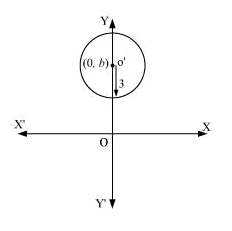Differentiating equation $$\left( 1 \right)$$ with respect to $$x$$, we get:

\begin{align}&2x + 2\left( {y - b} \right)y' = 0\\& \Rightarrow \; \left( {y - b} \right).y' = - x\\& \Rightarrow \; \left( {y - b} \right) = \frac{{ - x}}{{y'}}\end{align}

Substituting this value in equation $$\left( 1 \right)$$, we get:

\begin{align}&{x^2} + {\left( {\frac{{ - x}}{{y'}}} \right)^2} = 9\\& \Rightarrow \; {x^2}\left[ {1 + \frac{1}{{{{\left( {y'} \right)}^2}}}} \right] = 9\\& \Rightarrow \; {x^2}\left[ {{{\left( {y'} \right)}^2} + 1} \right] = 9{\left( {y'} \right)^2}\\ &\Rightarrow \; \left( {{x^2} - 9} \right){\left( {y'} \right)^2} + {x^2} = 0\end{align}

## Chapter 9 Ex.9.3 Question 11

Which of the following differential equations has $$y = {c_1}{e^x} + {c_2}{e^{ - x}}$$ as the general solution?

(A) $$\frac{{{d^2}y}}{{d{x^2}}} + y = 0$$

(B) $$\frac{{{d^2}y}}{{d{x^2}}} + y = 0$$

(C) $$\frac{{{d^2}y}}{{d{x^2}}} + 1 = 0$$

(D) $$\frac{{{d^2}y}}{{d{x^2}}} - 1 = 0$$

### Solution

\begin{align}&y = {c_1}{e^x} + {c_2}{e^{ - x}}\\ &\Rightarrow \; \frac{{dy}}{{dx}} = {c_1}{e^x} - {c_2}{e^{ - x}}\\ &\Rightarrow \; \frac{{{d^2}y}}{{d{x^2}}} = {c_1}{e^x} + {c_2}{e^{ - x}}\\& \Rightarrow \; \frac{{{d^2}y}}{{d{x^2}}} = y\\& \Rightarrow \; \frac{{{d^2}y}}{{d{x^2}}} - y = 0\end{align}

Thus, the correct option is B.

## Chapter 9 Ex.9.3 Question 12

Which of the following differential equations has $$y = x$$ as one of its particular solution?

(A) $$\frac{{{d^2}y}}{{d{x^2}}} - {x^2}\frac{{dy}}{{dx}} + xy = x$$

(B) $$\frac{{{d^2}y}}{{d{x^2}}} + x\frac{{dy}}{{dx}} + xy = x$$

(C) $$\frac{{{d^2}y}}{{d{x^2}}} - {x^2}\frac{{dy}}{{dx}} + xy = 0$$

(D) $$\frac{{{d^2}y}}{{d{x^2}}} + x\frac{{dy}}{{dx}} + xy = 0$$

### Solution

\begin{align}\frac{{dy}}{{dx}} &= 1\\\frac{{{d^2}y}}{{d{x^2}}} &= 0\end{align}

Therefore,

\begin{align}\frac{{{d^2}y}}{{d{x^2}}} - {x^2}\frac{{dy}}{{dx}} + xy &= 0 - {x^2}.1 + x.x\\ &= - {x^2} + {x^2}\\& = 0\end{align}

Thus, the correct option is C.

Instant doubt clearing with Cuemath Advanced Math Program